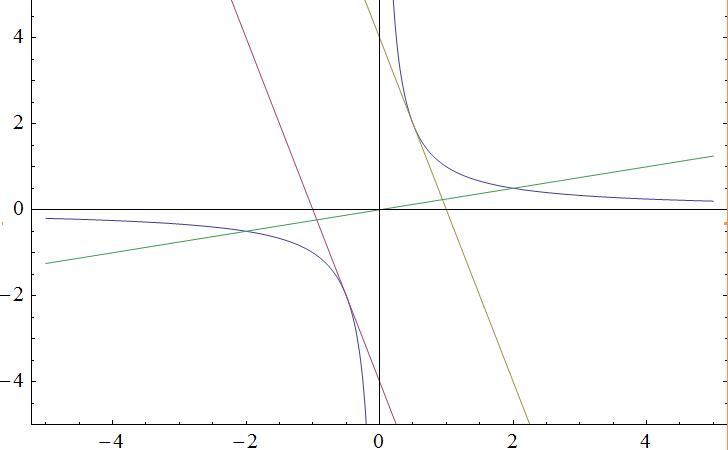# Determining slope of the tangent line.

fghtffyrdmns

## Homework Statement

I've never seen such a problem before: Find the two straight lines that are perpendicular to $$y=0.25x$$ and tanget to the curve $$f(x)=1/x$$

## Homework Equations

$$y=0.25x$$ ; $$f(x)=1/x$$

## The Attempt at a Solution

Using the power rule, I found the derivatives of: $$f(x)=-x^{-2}$$ and $$y=0.25$$

But I am not sure what I do now? Do I find the slope of the equations? Do I equate y=f(x)?

Last edited:

## Answers and Replies

Gregg
If they are perpendicular to y=x/4 then the slope of the line is what?

Now, to find a tangent to y=1/x.

you need the equation for the straight line:

$$y-y_0 = (x-x_0)f'(x_0)$$

what x0 is required to for the desired slope?

fghtffyrdmns
Is it -4?

I'm confused already :(.

fghtffyrdmns
Any help anyone? :(

Gregg
All you have to do is not get mixed up, we need a line that is tangent to 1/x. It also needs to be perpendicular to that line you described.So, to get a line that is normal to x/4, you do need a line that has a gradient or slope of -4.

$$y-y_0 = (x-x_0)m$$

First of all in order for the line to be tangent to 1/x it needs to parallel to it at a given point x0. The slope of f(x) is, as you say -1/x^2. The line is going to look like this:

$$y-y_0 = (x-x_0)f'(x_0)$$

and

$$f'(x_0)=-4$$

Get the x0s then find y0 and plug them into the line equation.

fghtffyrdmns
"the x0s then find y0"

What are these? What are the equations that you come up with?Gregg
Well the general equation for the line is going to take the form y=mx+c, in order to get that equation you need the slope (m) and a point on the line, which will be (x0), y0. For example if I knew my slope was 3 and that a point on my curve was (1,2) then I can work out that the equation for line is:

$$y-2=3(x-1), y=3x-1$$

The same is with this problem with have here, we need to work out at what point the slope is -4 don't we. The derivative of the curve is -1/x^2.

$$\frac{1}{x_0^2} = 4 \Rightarrow x_0 = \pm \frac{1}{2}$$

So you have two. Now all you do is work out the corresponding y values and use the equation for a straight line to work it out.

fghtffyrdmns
I figred out that the functions cross at 2 and -2. I can't use these points?

Gregg
Just find out what value -1/x^2 = -4, that's x1. (there's two of them)

Then plug it into y=1/x to get the y1. (there's two of them)

Then plug it into

y-y1=-4(x-x1)

and you will get 2 lines.

fghtffyrdmns
Okay, I got +/- 1/2 which gets me +/- 2

Now I'm confused which is x and which is y :/

Bohrok
You have two x values; plug them into f(x) = 1/x and you'll get the y values of the coordinates of the points tangent to 1/x. The plug them into the line equation y = mx + b, solve for b, then get the two equations of the tangent lines.

Gregg
x=-1/2
so what is y at this point, 1/(-1/2) = -2
So the corresponding y for -1/2 is -2. you can do the rest?

fghtffyrdmns
Alright. I got y=-4x-4 and y=-4x+4

Are these correct?

Bohrok
They are correct. You should try graphing on a calculator or by hand, or look for an online graphing calculator. 1/x should be easy to graph by hand.

GreggRed is -4x-4
Yellow is -4x+4
Green is x/4
Blue is 1/x

Nice!

fghtffyrdmns
Thank you guys :)!